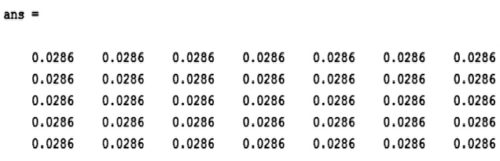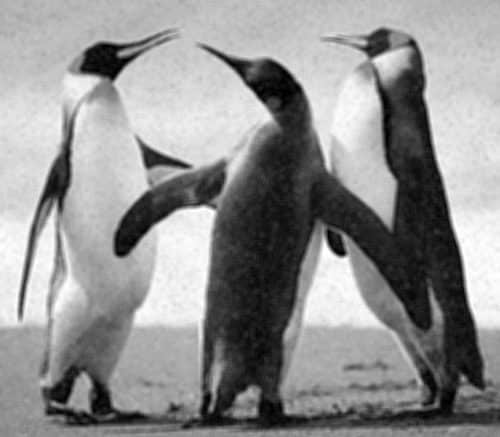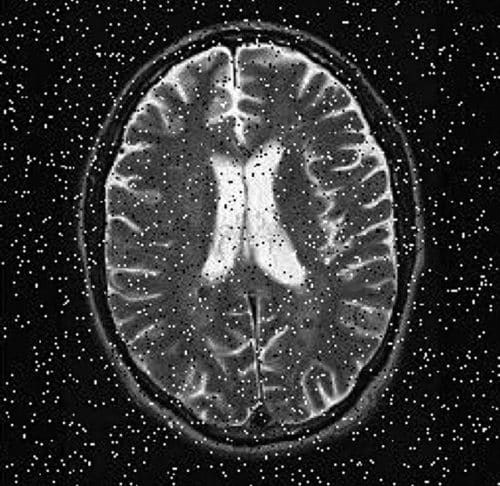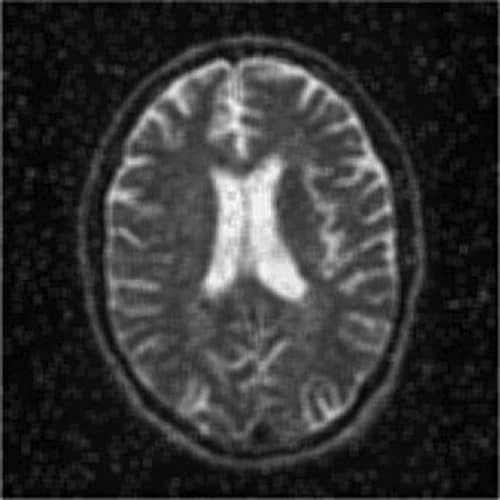# Image Processing Using MATLAB: Spatial Filtering (Part 3 of 4)

Dr Anil Kumar Maini is former director, Laser Science and Technology Centre, a premier laser and optoelectronics R&D laboratory of DRDO of Ministry of Defence

30724

2. ‘symmetric’. The boundaries of the input array (image) are extended by mirror-reflecting the image across its border.

3. ‘replicate’. The boundaries of the input array (image) are extended by replicating the values nearest to the image border.

4. ‘circular’. The boundaries of the input array (image) are extended by implicitly assuming the input array is periodic, that is, treating the image as one period of a 2D periodic function.

As regards size_options, there are two size options for the resulting image: ‘full’ (output image is the full filtered result, that is, the size of the extended/padded image) or ‘same’ (output image is of the same size as input image), which is the default.

‘g’ is the output image.

Correlation. Correlation is the same as convolution without mirroring (flipping) of the mask before the sums of products are computed. The difference between using correlation and convolution in 2D neighbourhood processing operations is often irrelevant because many popular masks used in image processing are symmetrical around the origin.

## Linear low-pass filters

The averaging filter is a linear LPF implemented using ‘average’ option in the fspecial function. The command given below produces an averaging filter of size 5×7:

fspecial(‘average’, [5,7])

The output of this command in MATLAB is:The code given below applies an averaging filter of dimensions 3×3 to the image Penguins_grey.jpg:

A = imread(‘Penguins_grey.jpg’);
B = fspecial(‘average’);
C = filter2(B,A);
figure, imshow(A),figure, imshow(C/255)

Matrix B is a 3×3 matrix with each matrix element having a value of 0.111. Matrix C has data of type ‘double.’ We can divide it by 255 to obtain a matrix with values in the 0-1 range for use with imshow function. Both the original image and the filtered image are shown in Figs 1 and 2, respectively.Fig. 1: Original imageFig. 2: Image using averaging filter of dimensions 3×3

As you can see from the filtered image, the averaging filter blurs the image and the edges in the image are less distinct than in the original image. Larger the size of the averaging filter, more is the blurring effect. The code given below shows the averaging filter operation using an average filter of 9×9:

A = imread(‘Penguins_grey.jpg’);
B = fspecial(‘average’,[9,9]);
C = filter2(B,A);
figure, imshow(A),figure, imshow(C/255)

Fig. 3 shows the filtered image.Fig. 3: Image using an average filter of 9×9

Averaging filter is an arithmetic mean filter. Other types of mean filters include geometric mean filters, harmonic mean filters and contra-harmonic mean filters. Mean filter is the simplest and the most widely used spatial smoothing filter.

The averaging filter operates on an mxn sliding window by calculating the average of all pixel values within the window and replacing the centre pixel value in the destination image with the result.

As mentioned above, the averaging filter causes the blurring effect in the image, proportional to the window size. This helps in reduction of noise, especially Gaussian or uniform noise. As an example, let us add salt and pepper noise to the Penguins_grey.jpg image and filter it using the averaging filter. Salt and pepper noise causes very bright (salt) and very dark (pepper) isolated spots in an image.

The command for adding salt and pepper noise to an image is:

A = imread(‘Penguins_grey.jpg’);
Noise_snp=imnoise(A,’salt & pepper’);
imshow(Noise_snp)

Fig. 4 shows the Penguins_grey.jpg image with salt and pepper noise added.Fig. 4: Image with salt and pepper noise added

Following commands remove the salt and pepper noise from Fig. 4:

>> B = fspecial(‘average’, [9,9]);
>> C = filter2(B,Noise_snp);
>>imshow(C/255)Fig. 5: The resultant image with salt and pepper noise removed

Fig. 5 shows the resultant image. As you can see, the image in Fig. 5 is blurred but the salt and pepper noise has been removed. Fig. 6 shows another image (MRI image) with salt and pepper noise and Fig. 7 shows the image after performing the following operations:

>> a=imread(‘MRI_snp.jpg’);
>>imshow(a)
>> B = fspecial(‘average’, [9,9]);
>> C = filter2(B,a);
>>imshow(C/255)Fig. 6: Another image (MRI image) with salt and pepper noiseFig. 7: The image in Fig. 6 after noise removal

A variation of the arithmetic mean filter is the geometric mean filter. It is primarily used on images with Gaussian noise. This filter is known to retain image detail better than the arithmetic mean filter.

Gaussian filters are another type of linear filter. These are low-pass filters, based on the Gaussian probability distribution function as given below:

ƒ(x)=e–x2/2σ2

where ‘σ’ is the standard deviation.

Gaussian filters have a blurring effect which looks very similar to that produced by neighbourhood averaging.

The following commands apply Gaussian filter to the image Penguins_grey.jpg:

>> A = imread(‘Penguins_grey.jpg’);
>> G1=fspecial(‘gaussian’,[11,11]);
>> G1image=filter2(G1,A)/256;
>>imshow(G1image)

The numbers within the square brackets give the size of the filter, the default value of which is 3×3. The final parameter is the standard deviation. If any value is not specified, a default value of 0.5 is assumed. In the command given above, since the standard deviation parameter is not specified, default value of 0.5 is taken.Fig. 8: Gaussian filtered image with default standard deviation value of 0.5

Fig. 8 shows a Gaussian filtered image with standard deviation value taken as 0.5.Fig. 9: Gaussian filtered image with standard deviation specified as 5

When the standard deviation is specified to be 5, as shown in the command (G1=fspecial(‘gaussian’,[11,11],5);), the resultant image is as shown in Fig. 9.

#### 2 COMMENTS

1.reaz

where is part 4?

•EFY Team

Thank you for your comment. We have updated the article with 4th part link. You can access the 4th article here.